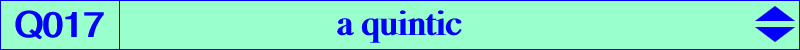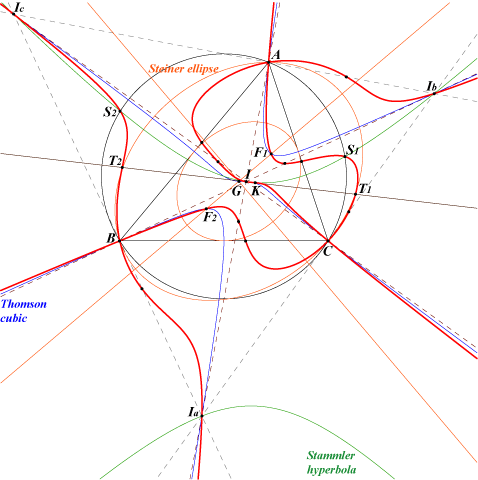Click on the link to download a text file.X(1), X(6) A, B, C (double) Ia, Ib, Ic : excenters vertices of the cevian triangle of K 4 foci of the Steiner in-ellipse (two real F1, F2) 4 intersections of the Stammler hyperbola and the circumcircle (two real S1, S2) 2 intersections T1, T2 of the line GK with the Steiner ellipseLet P be a point and let G', G" be the centroids of the pedal and antipedal triangles of P respectively. P, G', G" are collinear if and only if P lies on the quintic Q017 or on the line at infinity. Q017 has three singularities at A, B, C and contains the in/excenters. The "fifth" points on the bisectors at A are A5 = a^2 : 2b(b+c) : 2c(b+c) on AI and A5' = a^2 : 2b(b-c) : -2c(b-c) on IbIc. These points are collinear with the midpoint of BC. The "fifth" point on the symmedian AK is -2a^2 : b^2 : c^2. K is a flex with inflexional tangent GK, the tangent at K to the Stammler hyperbola.